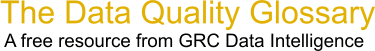Choose Index below for a list of all words and phrases defined in this glossary.

# Quantile

index | Index

## Quantile - definition(s)

quantile - [statistics] In a data distribution, a value representing a class break, where classes contain approximately equal numbers of observations. The p-th quantile, where p is between 0 and 1, is that value that has a proportion p of the data below the value. For theoretical distributions, the p-th quantile is the value that has p probability below the value.

[Category=Geospatial ]

Source: esri, 22 July 2012 10:26:50, http://support.esri.com/en/knowledgebase/GISDictionary/term/abbreviationData Quality Glossary.  A free resource from GRC Data Intelligence. For comments, questions or feedback: dqglossary@grcdi.nl